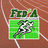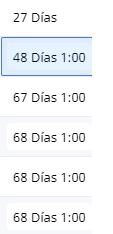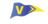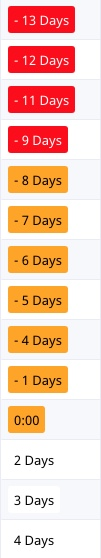### Remaining to a date & styleHi. I have this:

`let Countdown := 'Data d''inici' - today();`
`styled(text(Countdown), if Countdown = -1 then`
`"red"`
`else`
`if Countdown < 0 then "Orange" else "white" end`
`end)`

I want to format the f(x) like this: if 8 days remaining, color red. If 3 days remaining, color orange. And more options.

and how to avoid this? I just want the days and not the "1:00"ThanksTry this:
let x := date(ceil(number(Date) / 86400000) * 86400000);
let y := date(ceil(number(today()) / 86400000) * 86400000);
let countDown := x - y;
if countDown = -1 then
styled(text(day(x)), "Red")
else
if countDown < 0 then
styled(text(day(x)), "Orange")
else
styled(text(day(x)), "White")
end
end
end

There were two problems with your formula – I don't think you can run an if then statement within the styled() function (although I didn't try it!). The other problem is that ninox handles dates very in an odd way, which means there is often a bit of time (in your case, 1 hour) in the date or today() function. The formula at the start of the script deals with this.Try this:
let x := date(ceil(number(Date) / 86400000) * 86400000);
let y := date(ceil(number(today()) / 86400000) * 86400000);
let countDown := x - y;
if countDown = -1 then
styled(text(day(x)), "Red")
else
if countDown < 0 then
styled(text(day(x)), "Orange")
else
styled(text(day(x)), "White")
end
end
end

There were two problems with your formula – I don't think you can run an if then statement within the styled() function (although I didn't try it!). The other problem is that ninox handles dates very in an odd way, which means there is often a bit of time (in your case, 1 hour) in the date or today() function. The formula at the start of the script deals with this.Sorry – change x to countDown in the output!And the day() function is an error – this should be it!

let x := date(ceil(number(Date) / 86400000) * 86400000);
let y := date(ceil(number(today()) / 86400000) * 86400000);
let countDown := x - y;
if countDown = -1 then
styled(text(countDown), "Red")
else
if countDown < 0 then
styled(text(countDown), "Orange")
else
styled(text(countDown), "White")
end
end
endThanks for the reply! But not working. Your code sends me the same result as mine (no color and 1:00 included).

Thanks againStrange. Having rechecked, mine shows everything correctly (with colour) except -1. Are you using the App or Web version?
Dates are a real pain in Ninox.....If I come up with a solution I'll let you know..Ok –this code is giving me the output in the image:

let x := date(ceil(number(Date) / 86400000) * 86400000);
let y := date(ceil(number(today()) / 86400000) * 86400000);
let countDown := x - y;
if countDown < -(86400000 * 8) then
styled(text(countDown), "Red")
else
if countDown <= 0 then
styled(text(countDown), "Orange")
else
styled(text(countDown), "White")
end
end

I realised that Ninox is looking at the millisecond value of a day – so (8*86400000) for 8 days etc.) Hope this works for you – you should be able to modify to give you further variations.I'm using the web app (Windows 10, Chrome). And I don't know why the new code does no work too...